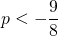# Solution assignment 09 Equation with fractions

### Assignment 9

For which value(s) of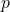does the following equation have no solutions: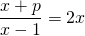### Solution

It seems that there is only a fraction in the left-hand side, but this is not the case. We can write: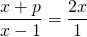Cross-multiplication yields: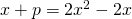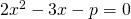This quadratic equation has no solution if its discriminant is less than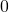is: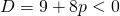Thus the equation has no solutions if: